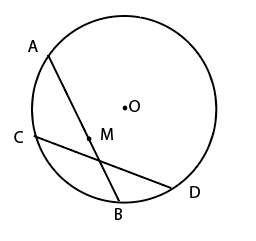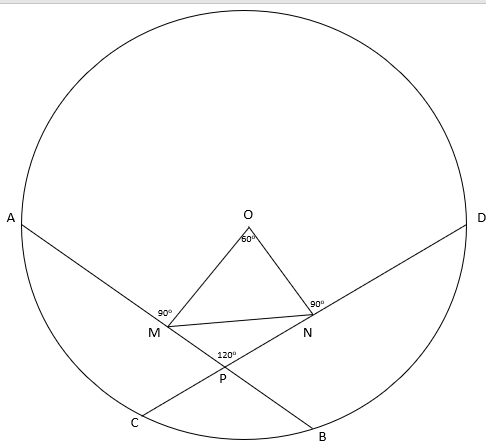Question 19

# As shown in the diagram, Ram started from point A along the chord AB and Shyam started from D along the chord DC. AB and DC are two equal chords. Ram and Shyam move with the same speed and started at the same time. After some time, Ram reached "M" which is the midpoint of AB. Ram and Shyam now make an angle $$60^{0}$$ at the centre O. If the distance between Ram and Shyam is 6 m now,then how much distance each will walk before they meet each other ?Solution

Using all the information in the question, the given figure becomes as shown below.Since the perpendiculars from centre to chords of equal lengths are always equal, $$\triangle$$OMN becomes an equilateral triangle with MN = 6 m.

In $$\triangle$$MNP, PM = PN  [by symmetry]

Thus, using the cosine rule,

$$MN^2=MP^2+NP^2-2\times MP\times NP\times cos(120)$$

$$36=MP^2+MP^2+MP^2$$

$$MP^2=12$$

$$MP=2\sqrt 3$$

Thus, each of them has to travel a distance of $$2\sqrt 3$$ m in order to meet each other.

Hence, the answer is option C.